# interrupts.c File Reference

`#include "lpc2xxx.h"`
`#include "interrupts.h"`
`#include "type.h"`
`#include "armVIC.h"`
`#include "types.h"`
Include dependency graph for interrupts.c: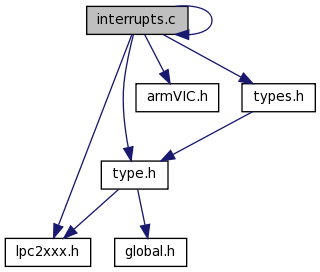This graph shows which files directly or indirectly include this file:## Functions

void timer_ISR (void)
void timer2_ISR (void)
void timer2_init (void)
void timer2_done (void)
void timer_init (void)

## Variables

UINT32 g_tick = 0
UINT32 g_tickP027 = 0

## Function Documentation

 void timer2_done ( void )

References PINSEL1, and T2TCR.

Referenced by Idle().

Here is the caller graph for this function:void timer2_init ( void )

Referenced by Idle(), and main().

Here is the call graph for this function:Here is the caller graph for this function: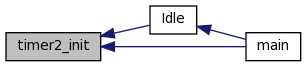void timer2_ISR ( void )

References g_tickP027, ISR_ENTRY, ISR_EXIT, T2IR, T2TCR, and VICVectAddr.

Referenced by timer2_init().

Here is the caller graph for this function:void timer_init ( void )

References T0CTCR, T0MCR, T0MR0, T0TCR, timer_ISR(), VICIntEnable, VICVectAddr0, and VICVectCntl0.

Referenced by main().

Here is the call graph for this function: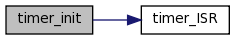Here is the caller graph for this function: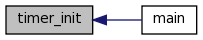void timer_ISR ( void )

References g_tick, ISR_ENTRY, ISR_EXIT, T0IR, and VICVectAddr.

Referenced by timer_init().

Here is the caller graph for this function: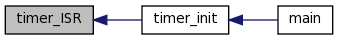## Variable Documentation

 UINT32 g_tick = 0

Referenced by GetTickCount(), and timer_ISR().

 UINT32 g_tickP027 = 0

Referenced by timer2_ISR().

Generated on Wed Mar 23 11:08:38 2011 for Omnima LCD by1.6.3